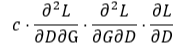# Numerical issue related to Hessian vector product

Hi, I found a problem that seems to be a numerical issue related to Hessian vector product. In the following code, I compare the difference between the result that obtained by Hessian vector product and the true value obtained by handcrafted derivatives. Pytorch 1.4.0, CPU

``````import torch
import torch.nn as nn

'''
return Hessian vector product
'''
allow_unused=True)
for i, p in enumerate(params):
else:
return hvp

class NetD(nn.Module):
def __init__(self):
super(NetD, self).__init__()
self.net = nn.Linear(2, 1)
self.weight_init()

def forward(self, x):
return self.net(x)

def weight_init(self):
self.net.weight.data = torch.Tensor([[1.0, 2.0]])
self.net.bias.data = torch.Tensor([-1.0])

class NetG(nn.Module):
def __init__(self):
super(NetG, self).__init__()
self.net = nn.Linear(1, 2)
self.weight_init()

def forward(self, x):
return self.net(x)

def weight_init(self):
self.net.weight.data = torch.Tensor([[3.0], [-1.0]])
self.net.bias.data = torch.Tensor([-4.0, 3.0])

lr = 0.01
factor = lr
D = NetD()
G = NetG()

z = torch.tensor([2.0])
real_x = torch.tensor([[3.0, 4.0]])
loss = D(G(z)) - D(real_x)
d_param = list(D.parameters())
g_param = list(G.parameters())

A_g = torch.tensor([[4.0 * factor, 0.0, 2.0 * factor, 0],
[0.0, 4.0 * factor, 0.0, 2.0 * factor],
[2.0 * factor, 0.0, factor, 0.0],
[0.0, 2.0 * factor, 0.0, factor]])
A_d = torch.tensor([[5.0 * factor, 0.0, 0.0],
[0.0, 5.0 * factor, 0.0],
[0.0, 0.0, 0.0]])
g_test = g_vec_d
d_test = d_vec_d

g_gt = g_vec_d.clone()
d_gt = d_vec_d.clone()
d_diffs = []
g_diffs = []
for i in range(1):
g_gt = torch.matmul(A_g, g_gt)
d_gt = torch.matmul(A_d, d_gt)

tmp1 = Hvp_vec(grad_d_vec, g_param, d_test, retain_graph=True).detach()
d_test = Hvp_vec(grad_g_vec, d_param, tmp1, retain_graph=True).detach().mul(factor)

tmp2 = Hvp_vec(grad_g_vec, d_param, g_test, retain_graph=True).detach()
g_test = Hvp_vec(grad_d_vec, g_param, tmp2, retain_graph=True).detach().mul(factor)
g_diffs.append((g_gt - g_test).tolist())
d_diffs.append((d_gt - d_test).tolist())

print('%.3f * D_dg * D_gd * grad_d :' % factor)
print(d_diffs)
print('%.3f * D_gd * D_dg * grad_g :' % factor)
print(g_diffs)
``````

The following figure explains the situation where the issue occurs. I build a toy GAN model here, which includes discriminator D and generator G. I try to compute this. When the factor © is bigger than 0.1, it’s consistent with the true value. But if the factor is 0.04, 0.01, there’s some difference between the result and true value.

c= 0.05

``````0.050 * D_dg * D_gd * grad_d :
[[0.0, 0.0, 0.0]]
0.050 * D_gd * D_dg * grad_g :
[[0.0, 0.0, 0.0, 0.0]]
``````

c= 0.04

``````0.040 * D_dg * D_gd * grad_d :
[[-1.4901161193847656e-08, -5.960464477539063e-08, 0.0]]
0.040 * D_gd * D_dg * grad_g :
[[0.0, 0.0, 0.0, 0.0]]
``````

c=0.01

``````0.010 * D_dg * D_gd * grad_d :
[[-3.725290298461914e-09, -1.4901161193847656e-08, 0.0]]
0.010 * D_gd * D_dg * grad_g :
[[0.0, 0.0, 0.0, 0.0]]
``````

Hi,

Is the error you’re seeing of the order of 1e-8?
Keep in mind that floating point number precision is only up to 1e-6/1e-7 and they are not commutative. So doing the same operation in a different order is expected to lead to such differences.

1 Like

Got it. Thx~